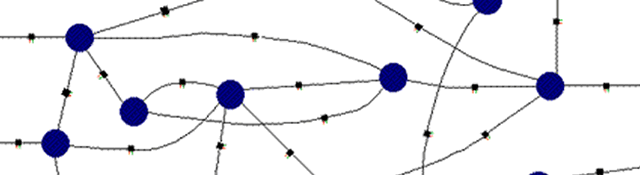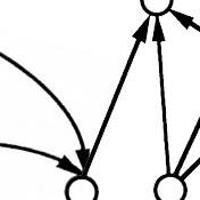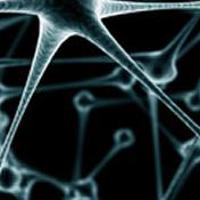## The modern artificial neuron

Modern artificial neurons have been derived from McCulloch-Pitts model, but with some changes. These changes allow us to create physical neural networks (using resistors, opamps, etc) relatively easily. Plus, these changes make the neurons much more versatile and can adapt to a wider range of applications.

## The changes

I assume you've gone through the post on McCulloch-Pitts model. So I relate the 3 changes that were made to make the modern neuron.

### Change 1: The inputs

In the McCulloch-Pitts model, the input could either be a zero or a one. In modern neurons, the inputs can take any value. It can be zero/one, or a decimal number.

### Change 2: The weights

In the original model, weights could be either a +1 or a -1, representing the excitatory or inhibitory inputs. In modern neurons, the idea of "excitatory" or "inhibitory" has been done away with. The weight is just a numeric value, which can have any value. This weight is just used to calculate the weighted-sum.

And changing these weights is what causes "learning" in neurons. We'll get to know about this later on.

### Change 3: Processing

In the original model, the neuron did no "processing". It just calculated the weighted sum, and then checked if the value is less than some pre-decided T or not. If it was, the output was made zero. Otherwise, it was made one.

In the new model, the neuron first calculates the weighted sum. And then takes this sum through a function. This function is called the "activation function". We'll go through some commonly used activation function in a later post. For now, just know that the sum is taken through a function (I mean a mathematical function, like sin(sum), etc).

And then the answer of this operation is checked if it is greater than T or not. This is optional though.

## Architectures

So now you know what a modern neuron does. If gets multiple inputs. Then it calculates the weighted sum of the inputs. Then it takes the sum through a mathematical function. And then optionally "thresholds" this sum (checks if it is less than T or not).

How is this useful, you ask? A single neuron itself isn't of much use. But with several neurons, we can create interesting things. You can create classifiers, predictors, recognizers, memories, and what not.

To do this, you need to arrange the several neurons in a certain fashion. You can't just say "Ok, I'll take 500 neurons, and connect them like this". A lot of research has been done on this topic to create useful neural networks. The way you interconnect neurons is called an architecture.

There exist several architectures. We'll go in detail with a lot of architectures, and create some really interesting things! Things that learn on their own. And things that "understand" what they're doing. And we'll also talk about how to make a particular architecture learn (each one has a different way).

#### Related posts#### 7 unique neural network architectures